## RS Aggarwal Class 8 Solutions Chapter 13 Time and Work Ex 13A

These Solutions are part of RS Aggarwal Solutions Class 8. Here we have given RS Aggarwal Solutions Class 8 Chapter 13 Time and Work Ex 13A.

Other Exercises

Question 1.
Solution:
Rajan’s one day’s work = $$\\ \frac { 1 }{ 24 }$$
Amit’s one day’s work = $$\\ \frac { 1 }{ 30 }$$Question 2.
Solution:
Ravi’s one hours = $$\\ \frac { 1 }{ 15 }$$
Both’s one day’s work = $$\\ \frac { 1 }{ 12 }$$or 6 hours, 40 minutes.

Question 3.
Solution:
A and B both’s one day’s work = $$\\ \frac { 1 }{ 6 }$$
A’s alone’s one day’s work = $$\\ \frac { 1 }{ 9 }$$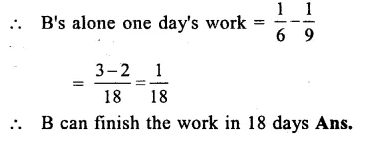Question 4.
Solution:
Raju and Siraj’s 1 hour work = $$\\ \frac { 1 }{ 6 }$$
Raju’s alone 1 hour work = $$\\ \frac { 1 }{ 15 }$$Question 5.
Solution:
A’s one day’s work = $$\\ \frac { 1}{ 10 }$$
B’s one day’s work = $$\\ \frac { 1 }{ 12 }$$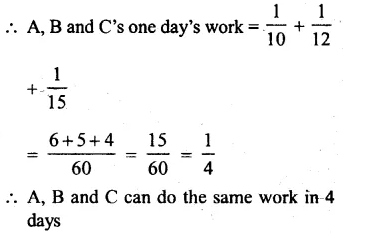Question 6.
Solution:
A’s 1 hour work = $$\\ \frac { 1 }{ 24 }$$
B’s 1 hour work = $$\\ \frac { 1 }{ 16 }$$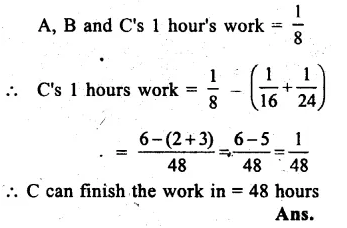Question 7.
Solution:
A,B and C’s 1 hr work = $$\\ \frac { 1 }{ 8 }$$
A’s 1 hour work = $$\\ \frac { 1 }{ 20 }$$
B’s 1 hour work = $$\\ \frac { 1 }{ 24 }$$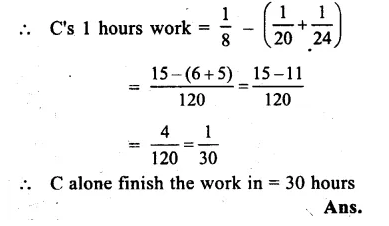Question 8.
Solution:
A’s one day’s work = $$\\ \frac { 1 }{ 16 }$$
B’s one days work = $$\\ \frac { 1 }{ 12 }$$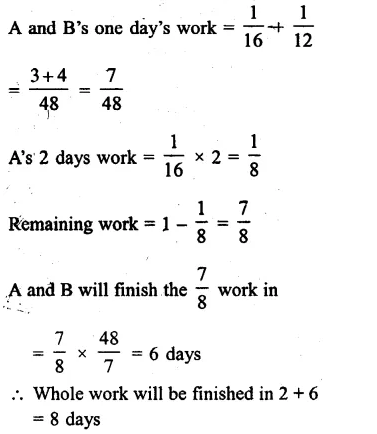Question 9.
Solution:
A’s 1 day’s work = $$\\ \frac { 1 }{ 14 }$$
B’s 1 day’s work = $$\\ \frac { 1 }{ 21 }$$Question 10.
Solution:
A can do $$\\ \frac { 2 }{ 3 }$$ work in = 16 days
A’s 1 days work = $$\\ \frac { 2 }{ 3 }$$ x $$\\ \frac { 1 }{ 16 }$$ = $$\\ \frac { 1 }{ 24 }$$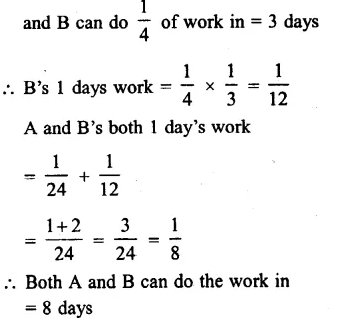Question 11.
Solution:
A’s one day’s work = $$\\ \frac { 1 }{ 15 }$$
B’s one day’s work = $$\\ \frac { 1 }{ 12 }$$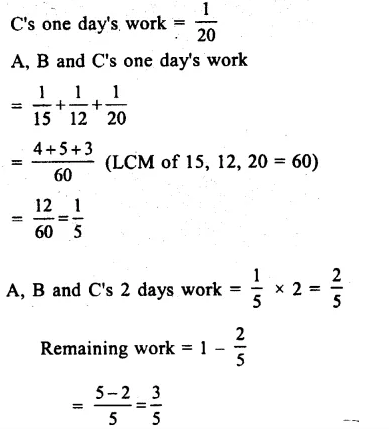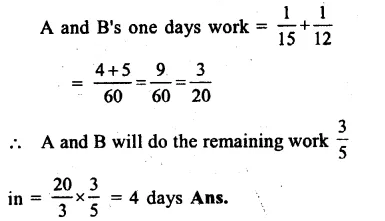Question 12.
Solution:
A and B’s one day’s work = $$\\ \frac { 1 }{ 18 }$$
B and C’s one day’s work = $$\\ \frac { 1 }{ 24 }$$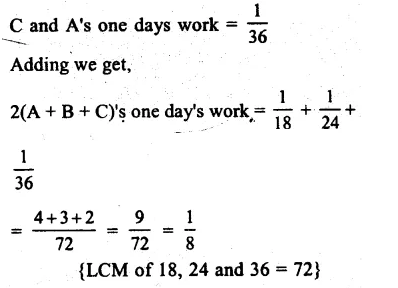A, B and C’s one days work = $$\frac { 1 }{ 2\times 2 }$$
= $$\\ \frac { 1 }{ 16 }$$
A, B and C can do the work in 16 days.

Question 13.
Solution:
A and B’s one days work = $$\\ \frac { 1 }{ 12 }$$
B and C’s one day’s work = $$\\ \frac { 1 }{ 15 }$$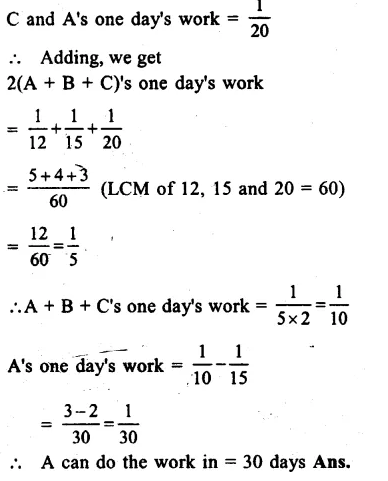Question 14.
Solution:
A’s one hr work =$$\\ \frac { 1 }{ 10 }$$
B’s one hr work = $$\\ \frac { 1 }{ 15 }$$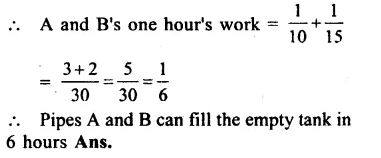Question 15.
Solution:
Pipe A’s one hour’s work for filling the tank = $$\\ \frac { 1 }{ 5 }$$
Pipe B’s one hour’s work for emptying = $$\\ \frac { 1 }{ 6 }$$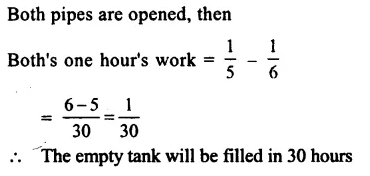Question 16.
Solution:
Tap A’s one hour’s work = $$\\ \frac { 1 }{ 6 }$$
Tap B’s one hour’s work = $$\\ \frac { 1 }{ 8 }$$
Tap C’s one hour’s work = $$\\ \frac { 1 }{ 12 }$$
A, B and C’s together one hour’s work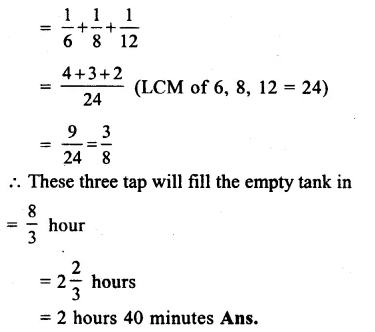Question 17.
Solution:
Inlet A’s 1 minutes work = $$\\ \frac { 1 }{ 12 }$$
Inlet B’s 1 minutes work = $$\\ \frac { 1 }{ 15 }$$Question 18.
Solution:
The inlet pipe’s 1 hour’s work = $$\\ \frac { 1 }{ 9 }$$
The leak and inlet’s 1 hours work = $$\\ \frac { 1 }{ 10 }$$
Leak’s 1 hour work = $$\frac { 1 }{ 9 } -\frac { 1 }{ 10 }$$
= $$\\ \frac { 10-9 }{ 90 }$$
= $$\\ \frac { 1 }{ 90 }$$
The leak can empty the cistern in = 90 hours Ans.

Question 19.
Solution:
Inlet pipe A’s one hour’s work = $$\\ \frac { 1 }{ 6 }$$
Inlet pipe B’s one hour’s work = $$\\ \frac { 1 }{ 8 }$$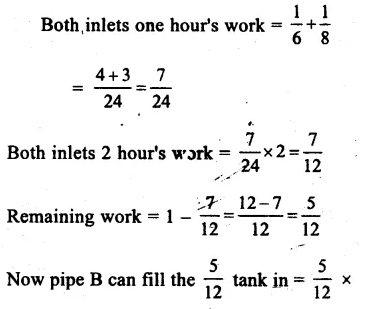Hope given RS Aggarwal Solutions Class 8 Chapter 13 Time and Work Ex 13A are helpful to complete your math homework.

If you have any doubts, please comment below. Learn Insta try to provide online math tutoring for you.